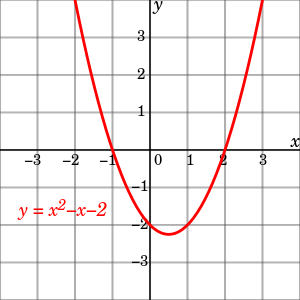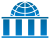# Dependent and independent variables

For dependent and independent random variables, see Independence (probability theory).

In mathematical modelling and statistical modelling, there are dependent and independent variables. The models investigate how the former depend on the latter. The dependent variables represent the output or outcome whose variation is being studied. The independent variables represent inputs or causes, i.e. potential reasons for variation. Models test or explain the effects that the independent variables have on the dependent variables. Sometimes, independent variables may be included for other reasons, such as for their potential confounding effect, without a wish to test their effect directly.In calculus, a function is typically graphed with the horizontal axis representing the independent variable and the vertical axis representing the dependent variable. In this function, y is the dependent variable and x is the independent variable.

## Mathematics

In mathematics, a function is a rule for taking an input (usually number or set of numbers) and providing an output (which is also usually a number). A symbol that stands for an arbitrary input is called an independent variable, while a symbol that stands for an arbitrary output is called a dependent variable. The most common symbol for the input is x, and the most common symbol for the output is y; the function itself is commonly written.

It is possible to have multiple independent variables and/or multiple dependent variables. For instance, in multivariable calculus, one often encounters functions of the form, where z is a dependent variable and x and y are independent variables. Functions with multiple outputs are often written as vector-valued functions.

In advanced mathematics, a function between a set X and a set Y is a subset of the Cartesian productsuch that every element of X appears in an ordered pair with exactly one element of Y. In this situation, a symbol representing an element of X may be called an independent variable and a symbol representing an element of Y may be called a dependent variable, such as when X is a manifold and the symbol x represents an arbitrary point in the manifold. However, many advanced textbooks do not distinguish between dependent and independent variables.

### Statistics

In an experiment, the dependent variable is the event expected to change when the independent variable is manipulated.

In data mining tools (for multivariate statistics and machine learning), the depending variable is assigned a role as target variable (or in some tools as label attribute), while a dependent variable may be assigned a role as regular variable. Known values for the target variable are provided for the training data set and test data set, but should be predicted for other data. The target variable is used in supervised learning algorithms but not in non-supervised learning.

### Modelling

In mathematical modelling, the dependent variable is studied to see if and how much it varies as the independent variables vary. In the simple stochastic linear modelthe termis the i th value of the dependent variable andis i th value of the independent variable. The termis known as the "error" and contains the variability of the dependent variable not explained by the independent variable.

With multiple independent variables, the expression is:, where n is the number of independent variables.

### Simulation

In simulation, the dependent variable is changed in response to changes in the independent variables.

## Statistics synonyms

Depending on the context, an independent variable is sometimes called a "predictor variable", "regressor", "controlled variable", "manipulated variable", "explanatory variable", "exposure variable" (see reliability theory), "risk factor" (see medical statistics), "feature" (in machine learning and pattern recognition) or "input variable."

Depending on the context, a dependent variable is sometimes called a "response variable", "regressand", "predicted variable", "measured variable", "explained variable", "experimental variable", "responding variable", "outcome variable", "output variable" or "label".

"Explanatory variable" is preferred by some authors over "independent variable" when the quantities treated as independent variables may not be statistically independent or independently manipulable by the researcher. If the independent variable is referred to as an "explanatory variable" then the term "response variable" is preferred by some authors for the dependent variable.

"Explained variable" is preferred by some authors over "dependent variable" when the quantities treated as "dependent variables" may not be statistically dependent. If the dependent variable is referred to as an "explained variable" then the term "predictor variable" is preferred by some authors for the independent variable.

Variables may also be referred to by their form: continuous, binary/dichotomous, nominal categorical, and ordinal categorical, among others.

## Other variables

A variable may be thought to alter the dependent or independent variables, but may not actually be the focus of the experiment. So that variable will be kept constant or monitored to try to minimise its effect on the experiment. Such variables may be designated as either a "controlled variable", "control variable", or "extraneous variable".

Extraneous variables, if included in a regression as independent variables, may aid a researcher with accurate response parameter estimation, prediction, and goodness of fit, but are not of substantive interest to the hypothesis under examination. For example, in a study examining the effect of post-secondary education on lifetime earnings, some extraneous variables might be gender, ethnicity, social class, genetics, intelligence, age, and so forth. A variable is extraneous only when it can be assumed (or shown) to influence the dependent variable. If included in a regression, it can improve the fit of the model. If it is excluded from the regression and if it has a non-zero covariance with one or more of the independent variables of interest, its omission will bias the regression's result for the effect of that independent variable of interest. This effect is called confounding or omitted variable bias; in these situations, design changes and/or statistical control is necessary.

Extraneous variables are often classified into three types:

1. Subject variables, which are the characteristics of the individuals being studied that might affect their actions. These variables include age, gender, health status, mood, background, etc.
2. Blocking variables or experimental variables are characteristics of the persons conducting the experiment which might influence how a person behaves. Gender, the presence of racial discrimination, language, or other factors may qualify as such variables.
3. Situational variables are features of the environment in which the study or research was conducted, which have a bearing on the outcome of the experiment in a negative way. Included are the air temperature, level of activity, lighting, and the time of day.

In modelling, variability that is not covered by the independent variable is designated byand is known as the "residual", "side effect", "error", "unexplained share", "residual variable", or "tolerance".

## Examples

• Effect of fertilizer on plant growth
In a study measuring the influence of different quantities of fertilizer on plant growth, the independent variable would be the amount of fertilizer used. The dependent variable would be the growth in height or mass of the plant. The controlled variables would be the type of plant, the type of fertilizer, the amount of sunlight the plant gets, the size of the pots, etc.
• Effect of drug dosage on symptom severity
In a study of how different doses of a drug affect the severity of symptoms, a researcher could compare the frequency and intensity of symptoms when different doses are administered. Here the independent variable is the dose and the dependent variable is the frequency/intensity of symptoms.
• Effect of temperature on pigmentation
In measuring the amount of color removed from beetroot samples at different temperatures, temperature is the independent variable and amount of pigment removed is the dependent variable.

## References

1. Hastings, Nancy Baxter. Workshop calculus: guided exploration with review. Vol. 2. Springer Science & Business Media, 1998. p. 31
2. Carlson, Robert. A concrete introduction to real analysis. CRC Press, 2006. p.183
3. Stewart, James. Calculus. Cengage Learning, 2011. Section 1.1
4. Anton, Howard, Irl C. Bivens, and Stephen Davis. Calculus Single Variable. John Wiley & Sons, 2012. Section 0.1
5. Larson, Ron, and Bruce Edwards. Calculus. Cengage Learning, 2009. Section 13.1
6. Hrbacek, Karel, and Thomas Jech. Introduction to Set Theory, Revised and Expanded. Vol. 220. Crc Press, 1999. p. 26
7. For instance, a Google Books search for "independent variable" on Mar 18, 2015 brought up 0 hits in the following advanced textbooks:
• Munkres, James R. Topology: a first course. Vol. 23. Englewood Cliffs, NJ: Prentice-Hall, 1975.
• Hungerford, Thomas. Abstract algebra: an introduction. Cengage Learning, 2012.
• Abbott, Stephen. Understanding analysis. Springer Science & Business Media, 2010.
8. Random House Webster's Unabridged Dictionary. Random House, Inc. 2001. Page 534, 971. ISBN 0-375-42566-7.
9. English Manual version 1.0 for RapidMiner 5.0, October 2013.
10. Dodge, Y. (2003) The Oxford Dictionary of Statistical Terms, OUP. ISBN 0-19-920613-9 (entry for "independent variable")
11. Dodge, Y. (2003) The Oxford Dictionary of Statistical Terms, OUP. ISBN 0-19-920613-9 (entry for "regression")
12. Everitt, B.S. (2002) Cambridge Dictionary of Statistics, CUP. ISBN 0-521-81099-X
13. Dodge, Y. (2003) The Oxford Dictionary of Statistical Terms, OUP. ISBN 0-19-920613-9
14. Ash Narayan Sah (2009) Data Analysis Using Microsoft Excel, New Delhi. ISBN 978-81-7446-716-4Wikiversity has learning materials about Independent variableWikiversity has learning materials about Dependent variable